Powers of z Next  |  Prev  |  Up  |  Top  |  Index  |  JOS Index  |  JOS Pubs  |  JOS Home  |  Search

### Powers of z

Choose any two complex numbers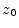and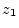, and form the sequence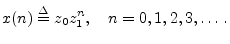(4.10)

What are the properties of this signal? Writing the complex numbers as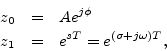we see that the signal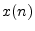is always a discrete-time generalized (exponentially enveloped) complex sinusoid: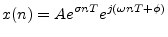Figure 4.17 shows a plot of a generalized (exponentially decaying,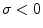) complex sinusoid versus time.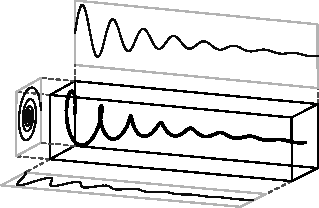Note that the left projection (onto the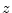plane) is a decaying spiral, the lower projection (real-part vs. time) is an exponentially decaying cosine, and the upper projection (imaginary-part vs. time) is an exponentially enveloped sine wave.

Next  |  Prev  |  Up  |  Top  |  Index  |  JOS Index  |  JOS Pubs  |  JOS Home  |  Search

[How to cite this work] [Order a printed hardcopy]